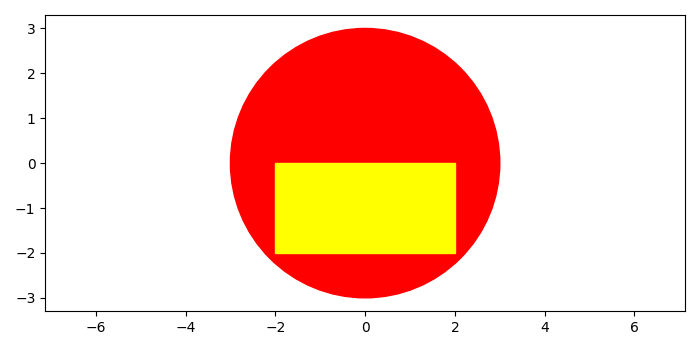# How to plot a rectangle inside a circle in Matplotlib?

To plot a rectangle inside a circle in matplotlib, we can take the following steps −

• Create a new figure or activate an existing figure using figure method.

• Add a subplot to the current axis.

• Make a rectangle and a circle instance using Rectangle() and Circle() class.

• Add a patch on the axes.

• Scale x and y axes using xlim() and ylim() methods.

• To display the figure, use show() method.

## Example

import matplotlib
from matplotlib import pyplot as plt, patches
plt.rcParams["figure.figsize"] = [7.00, 3.50]
plt.rcParams["figure.autolayout"] = True
fig = plt.figure()
rect1 = patches.Rectangle((-2, -2), 4, 2, color='yellow')
circle1 = matplotlib.patches.Circle((0, 0), radius=3, color='red')
plt.show()